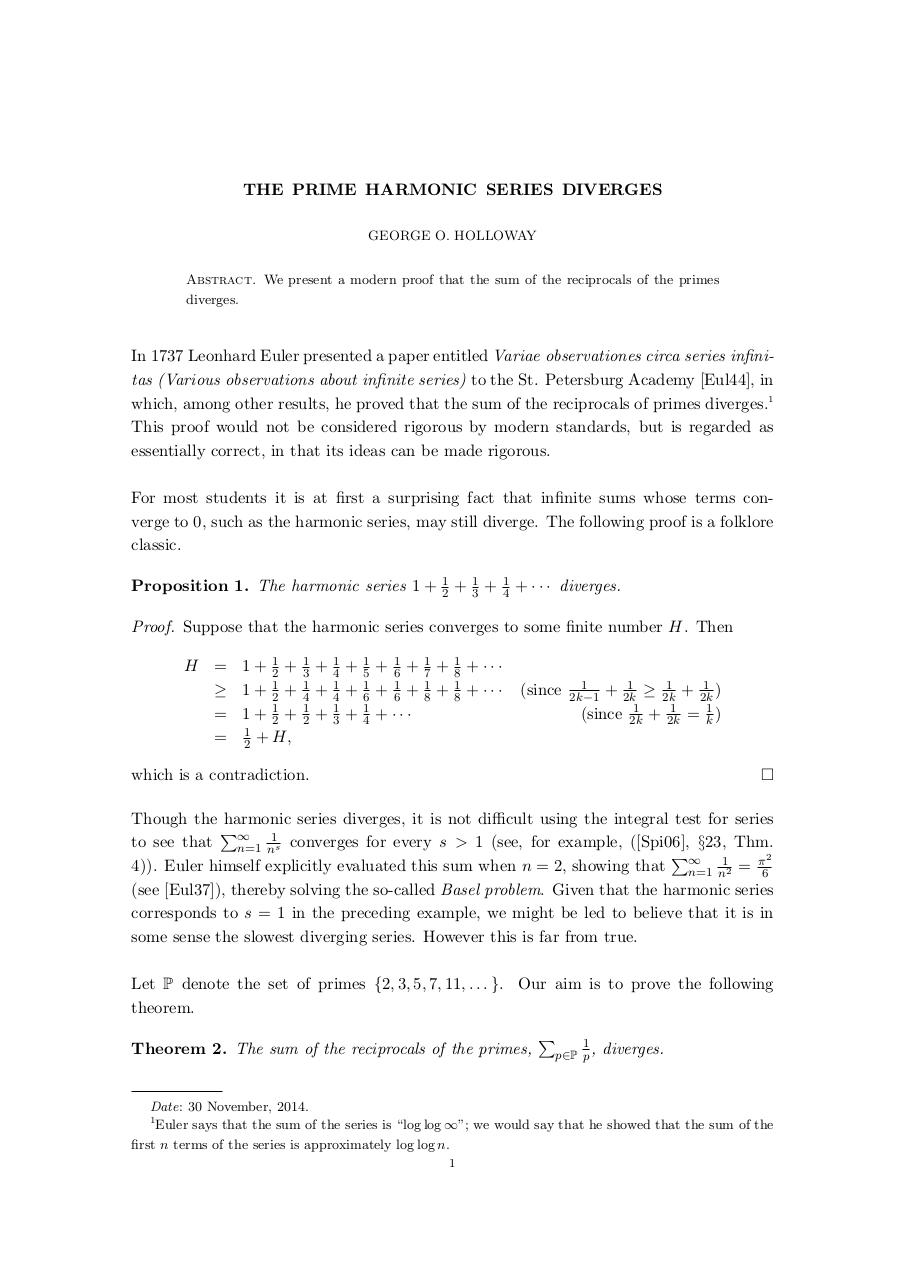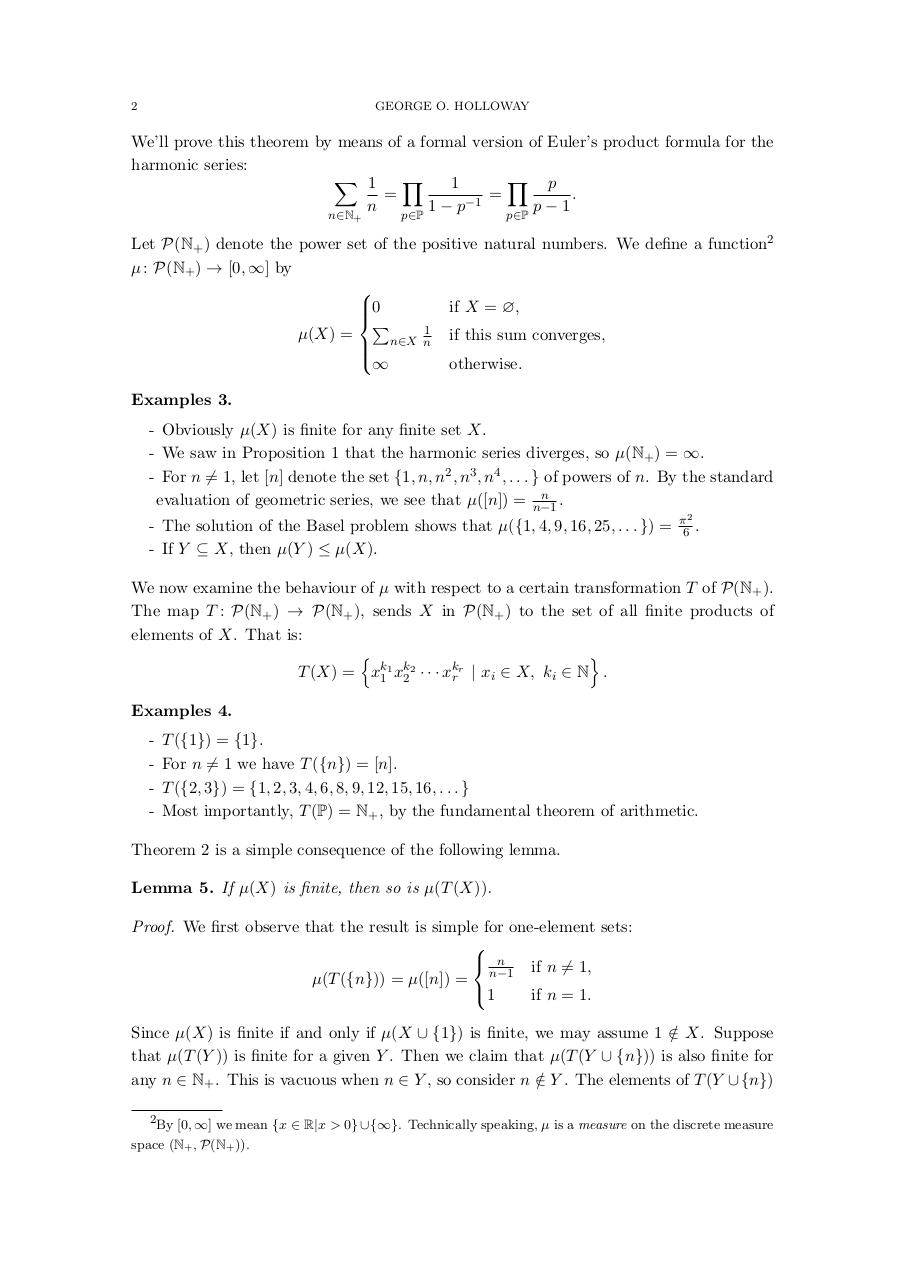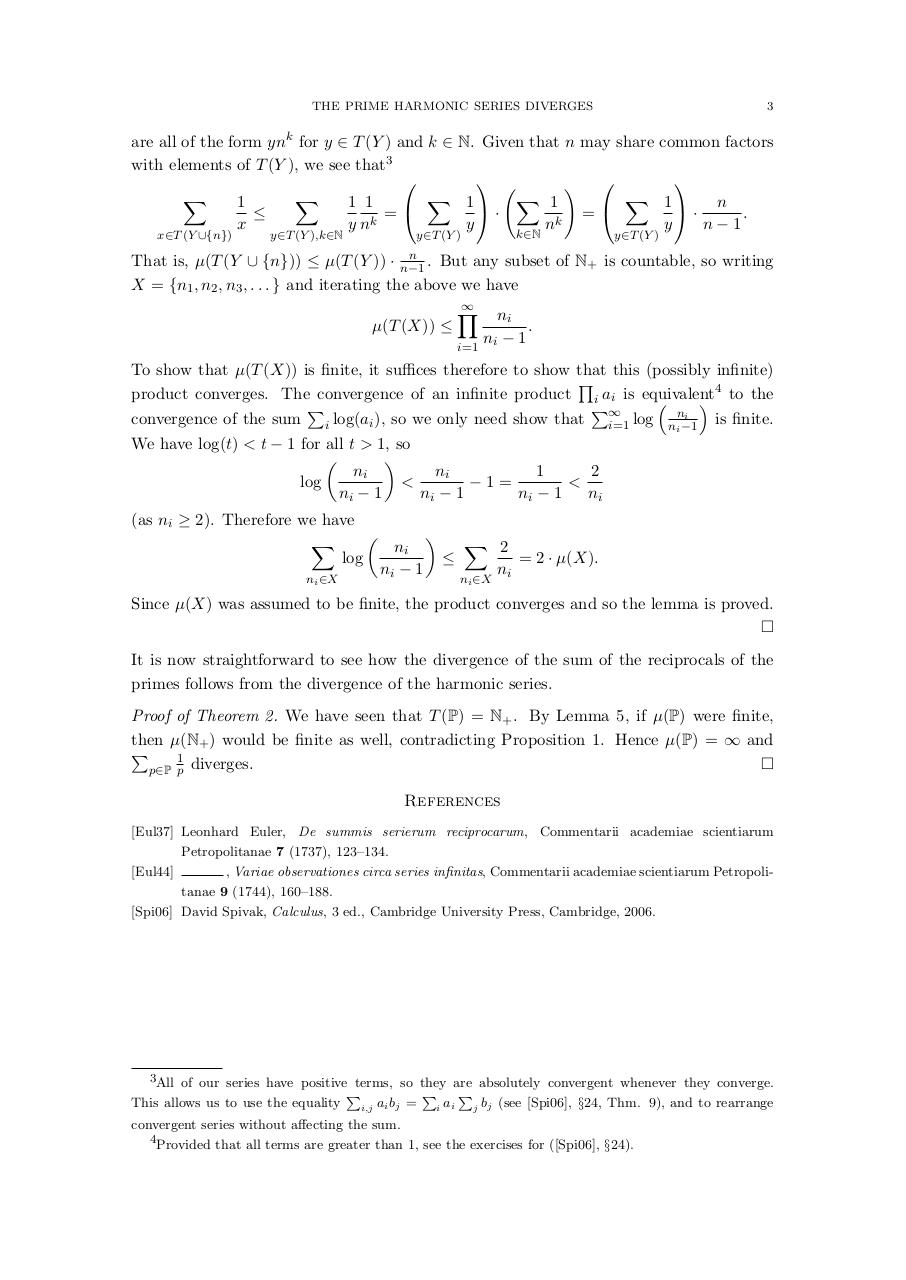# EulerPrimeRecips .pdf

### File information

Original filename: EulerPrimeRecips.pdf

This PDF 1.5 document has been generated by TeX / pdfTeX-1.40.16, and has been sent on pdf-archive.com on 14/07/2016 at 22:05, from IP address 86.173.x.x. The current document download page has been viewed 309 times.
File size: 220 KB (3 pages).
Privacy: public file

EulerPrimeRecips.pdf (PDF, 220 KB)

### Document preview

THE PRIME HARMONIC SERIES DIVERGES
GEORGE O. HOLLOWAY
Abstract. We present a modern proof that the sum of the reciprocals of the primes
diverges.

In 1737 Leonhard Euler presented a paper entitled Variae observationes circa series infinitas (Various observations about infinite series) to the St. Petersburg Academy [Eul44], in
which, among other results, he proved that the sum of the reciprocals of primes diverges.1
This proof would not be considered rigorous by modern standards, but is regarded as
essentially correct, in that its ideas can be made rigorous.
For most students it is at first a surprising fact that infinite sums whose terms converge to 0, such as the harmonic series, may still diverge. The following proof is a folklore
classic.
Proposition 1. The harmonic series 1 +

1
2

+

1
3

+

1
4

+ · · · diverges.

Proof. Suppose that the harmonic series converges to some finite number H. Then
H = 1 + 21 +
≥ 1 + 12 +
= 1 + 12 +
= 12 + H,

1
3
1
4
1
2

+
+
+

1
4
1
4
1
3

+
+
+

1
5
1
6
1
4

+ 16 +
+ 16 +
+ ···

1
7
1
8

+
+

1
8
1
8

+ ···
+ ···

(since

1
2k−1

1
1
1
+ 2k
≥ 2k
+ 2k
)
1
1
1
(since 2k + 2k = k )



Though the harmonic series diverges, it is not difficult using the integral test for series
P
1
to see that ∞
n=1 ns converges for every s &gt; 1 (see, for example, ([Spi06], §23, Thm.
P
1
π2
4)). Euler himself explicitly evaluated this sum when n = 2, showing that ∞
n=1 n2 = 6
(see [Eul37]), thereby solving the so-called Basel problem. Given that the harmonic series
corresponds to s = 1 in the preceding example, we might be led to believe that it is in
some sense the slowest diverging series. However this is far from true.
Let P denote the set of primes {2, 3, 5, 7, 11, . . . }. Our aim is to prove the following
theorem.
Theorem 2. The sum of the reciprocals of the primes,

P

1
p∈P p ,

diverges.

Date: 30 November, 2014.
1
Euler says that the sum of the series is “log log ∞”; we would say that he showed that the sum of the
first n terms of the series is approximately log log n.
1

2

GEORGE O. HOLLOWAY

We’ll prove this theorem by means of a formal version of Euler’s product formula for the
harmonic series:
X 1
Y
Y p
1
=
=
.
−1
n
1−p
p−1
n∈N+

p∈P

p∈P

Let P(N+ ) denote the power set of the positive natural numbers. We define a function2
µ : P(N+ ) → [0, ∞] by

if X = ∅,

0
P
1
µ(X) =
if this sum converges,
n∈X n

otherwise.
Examples 3.
- Obviously µ(X) is finite for any finite set X.
- We saw in Proposition 1 that the harmonic series diverges, so µ(N+ ) = ∞.
- For n 6= 1, let [n] denote the set {1, n, n2 , n3 , n4 , . . . } of powers of n. By the standard
n
evaluation of geometric series, we see that µ([n]) = n−1
.
- The solution of the Basel problem shows that µ({1, 4, 9, 16, 25, . . . }) =
- If Y ⊆ X, then µ(Y ) ≤ µ(X).

π2
6 .

We now examine the behaviour of µ with respect to a certain transformation T of P(N+ ).
The map T : P(N+ ) → P(N+ ), sends X in P(N+ ) to the set of all finite products of
elements of X. That is:
o
n
T (X) = xk11 xk22 · · · xkr r | xi ∈ X, ki ∈ N .
Examples 4.
-

T ({1}) = {1}.
For n 6= 1 we have T ({n}) = [n].
T ({2, 3}) = {1, 2, 3, 4, 6, 8, 9, 12, 15, 16, . . . }
Most importantly, T (P) = N+ , by the fundamental theorem of arithmetic.

Theorem 2 is a simple consequence of the following lemma.
Lemma 5. If µ(X) is finite, then so is µ(T (X)).
Proof. We first observe that the result is simple for one-element sets:

 n
if n 6= 1,
µ(T ({n})) = µ([n]) = n−1
1
if n = 1.
Since µ(X) is finite if and only if µ(X ∪ {1}) is finite, we may assume 1 ∈
/ X. Suppose
that µ(T (Y )) is finite for a given Y . Then we claim that µ(T (Y ∪ {n})) is also finite for
any n ∈ N+ . This is vacuous when n ∈ Y , so consider n ∈
/ Y . The elements of T (Y ∪ {n})
2By [0, ∞] we mean {x ∈ R|x &gt; 0}∪{∞}. Technically speaking, µ is a measure on the discrete measure

space (N+ , P(N+ )).

THE PRIME HARMONIC SERIES DIVERGES

3

are all of the form ynk for y ∈ T (Y ) and k ∈ N. Given that n may share common factors
with elements of T (Y ), we see that3

! 
X
X
X
X
X
1
1
1
1 1
n
1
=
=

·
·
.
x
y nk
y
y
n−1
nk
x∈T (Y ∪{n})

k∈N

y∈T (Y )

y∈T (Y ),k∈N

y∈T (Y )

n
n−1 .

But any subset of N+ is countable, so writing
That is, µ(T (Y ∪ {n})) ≤ µ(T (Y )) ·
X = {n1 , n2 , n3 , . . . } and iterating the above we have
µ(T (X)) ≤

Y
i=1

ni
.
ni − 1

To show that µ(T (X)) is finite, it suffices therefore to show that this (possibly infinite)
Q
4
product converges. The convergence of an infinite product i ai is equivalent

 to the
P
P∞
i
is finite.
convergence of the sum i log(ai ), so we only need show that i=1 log nin−1
We have log(t) &lt; t − 1 for all t &gt; 1, so


ni
ni
1
2
&lt;
log
−1=
&lt;
ni − 1
ni − 1
ni − 1
ni
(as ni ≥ 2). Therefore we have


X
X 2
ni
log

= 2 · µ(X).
ni − 1
ni
ni ∈X

ni ∈X

Since µ(X) was assumed to be finite, the product converges and so the lemma is proved.

It is now straightforward to see how the divergence of the sum of the reciprocals of the
primes follows from the divergence of the harmonic series.
Proof of Theorem 2. We have seen that T (P) = N+ . By Lemma 5, if µ(P) were finite,
then µ(N+ ) would be finite as well, contradicting Proposition 1. Hence µ(P) = ∞ and
P
1

p∈P p diverges.
References
[Eul37] Leonhard Euler, De summis serierum reciprocarum, Commentarii academiae scientiarum
Petropolitanae 7 (1737), 123–134.
[Eul44]
, Variae observationes circa series infinitas, Commentarii academiae scientiarum Petropolitanae 9 (1744), 160–188.
[Spi06] David Spivak, Calculus, 3 ed., Cambridge University Press, Cambridge, 2006.

3All of our series have positive terms, so they are absolutely convergent whenever they converge.

This allows us to use the equality

P

i,j

ai bj =

P

i

ai

P

j

bj (see [Spi06], §24, Thm. 9), and to rearrange

convergent series without affecting the sum.
4Provided that all terms are greater than 1, see the exercises for ([Spi06], §24).#### HTML Code

Copy the following HTML code to share your document on a Website or Blog

#### QR Code### Related keywords